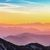QlikView App Dev

Discussion Board for collaboration related to QlikView App Development.

Announcements
QLIKWORLD LIVE! MAY 16 - 19TH, EARLY BIRD DISCOUNTS! REGISTER TODAY
cancel
Showing results for
Did you mean:Contributor

calculation

i have invoice \$70.00 and need to fine the usage of it but it have diff charges for diff level of unit usage,

1 unit to 10 unit charges are \$ 1.00/unit

11 unit to 20 unit charges are \$ 1.50/unit

21 unit to max unit Charges are \$2.00/unit

Labels (1)
• Calculation based total amount find the Usage

3 RepliesContributor II

Could you please be more clear ?Contributor

I have similar problem:

when I try to calculate sale = effective_price * quantity

however, it does not show the result of multiplication...Contributor
Author

Total amount \$ 70.00

I need find the max amount of each level diff unit  price first,

\$ 1.00 x 10 unit= \$10.00 -max amount 1

\$1.50 x 10 unit =\$15.00 -max amount 2

next i need to check is it my total amount is more than the max amount 1 or 2 or less than max amount 1 on above .

in this case it \$70.00 which is amount have exceeding both of max amount

1st step

First 10 unit of \$1.00/unit price=\$10.00

Total amount -Max amount of First 10 unit=Balance 1

\$70.00-\$10.00=\$60.00

2nd Step

Second 10 unit of \$1.50/unit price=\$15.00

Balance 1-Max amount of Second 10 unit=Balance 2

\$60.00-\$15.00= \$45

3rd Step

finally i have last unit price \$2.00 for the remaining Total amount, that mean my Balance 2 divide by \$2.00/unit Price i will get total usage for \$2.00/unit Price

Balance 2 / \$2.00

\$45.00/\$2.00= 22.5 unit usage

Finally result : Total unit Usage

1st step Unit Usage + 2nd step unit usage + 3rd step usage

=10 unit + 10 unit + 22.5 unit

Grand total = 42.5 unitCommunity Browser